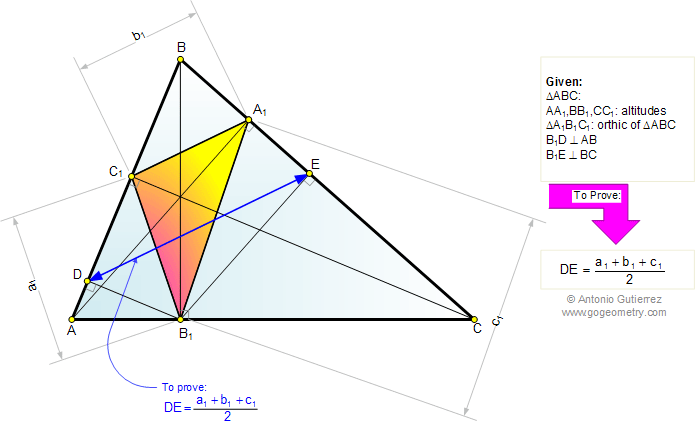# Online Geometry Problem 735: Triangle, Altitude, Perpendicular,  Semiperimeter of the Orthic Triangle, Congruence. Level: High School, Honors Geometry, College, Mathematics Education

 The figure shows a triangle ABC with the altitudes AA1, BB1, and CC1. If B1D is perpendicular to AB, and B1E is perpendicular to BC, prove that DE is equal to the semiperimeter of the triangle A1B1C1. Note: The triangle A1B1C1 is called the orthic triangle of the triangle ABC.Home | Search | Geometry | Problems | All Problems | Open Problems | Visual Index | 731-740 | Triangles | Altitude | Orthic Triangle | Perpendicular lines | Semiperimeter | Congruence | Email | Solution / comment | By Antonio Gutierrez